# Differentiable

Differentiable

In this mini-lesson, we will explore the world of differentiable functions. We will try answering questions like what is the meaning of differentiable, how to use derivative rules to find the function is differentiable, understand the importance of limits in derivative, and discover other interesting aspects of it. You can check out the interactive simulation to know more about the lesson and try your hand at solving a few interesting interactive questions at the end of the page.

You can think of the derivative of a function as its slope, just like mountains have a slope!

The slope of a mountain shows its steepness.Similarly, the slope of a function tells us how steep it is at those points.

In this short lesson, we will learn about differentiable functions.

So, let's start!

## Lesson Plan

 1 What Is Meant by Differentiable? 2 Important Notes on Differentiable 3 Solved Examples on Differentiable 4 Tips and Tricks on Differentiable 5 Interactive Questions on Differentiable

## What Is Meant by Differentiable?

### Differentiable Meaning

A function is said to be differentiable if the derivative of the function exists at all points in its domain.

Particularly, if a function $$f(x)$$ is differentiable at $$x=a$$, then $$f'(a)$$ exists in the domain.

Examples

The following table shows the list of some functions that are differentiable in $$\mathbb{R}$$.

Functions Examples
Polynomial functions $$f_{1}(x)=x^2+1$$, $$f_{2}(x)=x^3-x-9$$, $$f_{3}(x)=x^{100}$$
Transcendental functions $$f_{1}(x)=\cos{x}$$, $$f_{2}(x)=\sin{x}$$, $$f_{3}(x)=e^x$$

## How To Use Derivative Rules To Find Function Is Differentiable?

### Differentiability Formulas

Here are some derivative rules used to find the derivatives of a function.

 $(f \pm g)'=f' \pm g'$
 $(f g)'=f' g+f g'$
 $\left(\dfrac{f}{g}\right)'=\dfrac{f' g-f g'}{g^2}$

Example

Let's use the differentiability formulas to find the derivative of the function $$f(x)=(2x+1)^3$$.

\begin{align}\dfrac{df}{dx}&=\dfrac{d}{dx}(2x+1)^3\\&=\dfrac{d}{dx}(8x^3+12x^2+6x+1)\\&=24x^2+24x+6\\&=6(2x+1)^2\end{align}

## Why Limit Is Important to Know If the Function Is Differentiable Or Not?

Do you know there is an alternative way to determine if a function $$f(x)$$ is differentiable using the limits?

A function $$f(x)$$ is differentiable at the point $$x=a$$ if the following limit exists.

 $\lim_{h\rightarrow 0}\dfrac{f(c+h)-f(c)}{h}$

Example

Consider the absolute value function given by $$f(x)=|x|$$.

The graph of the function is shown below.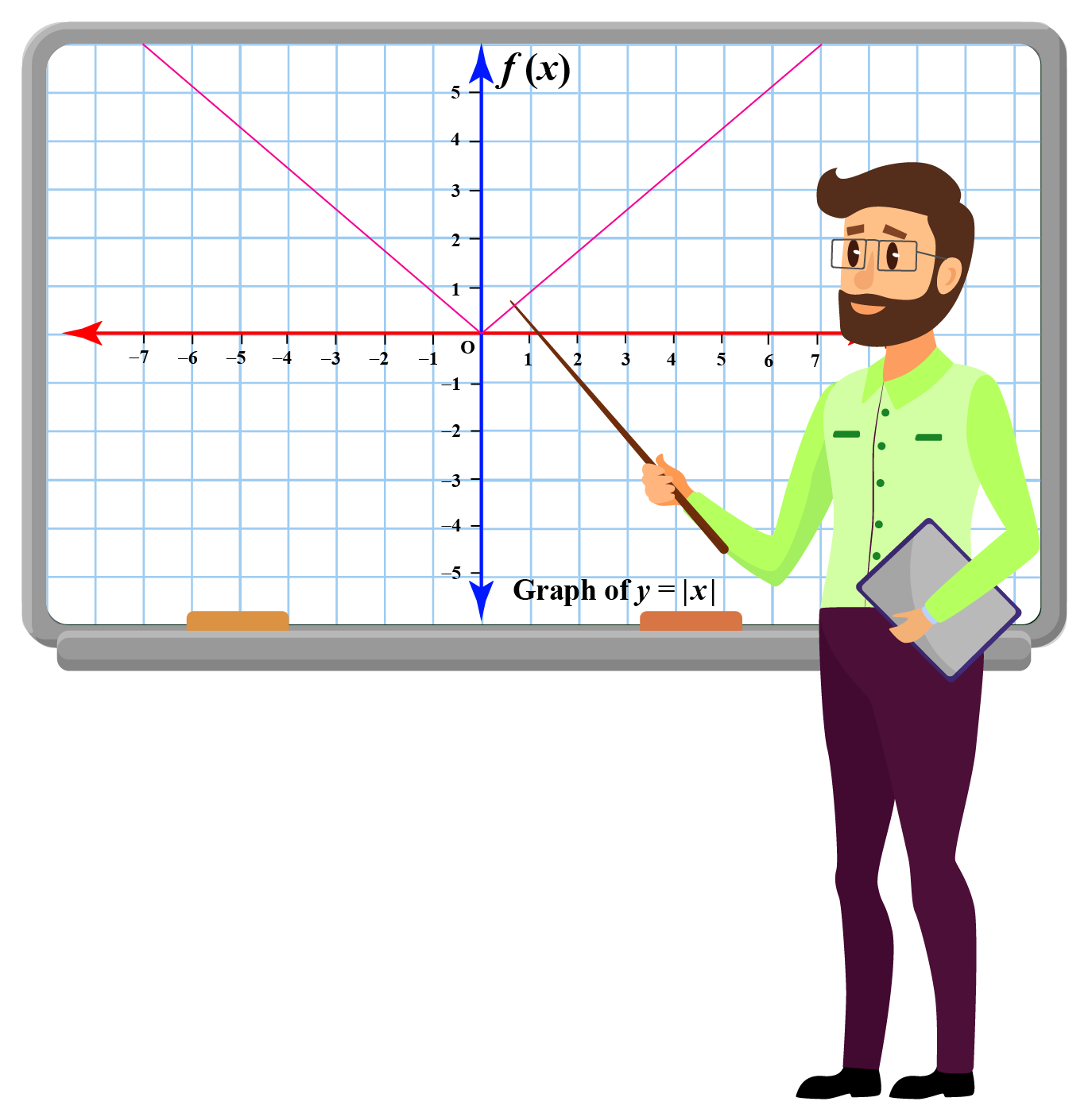We will determine if this function is differentiable at $$c=0$$ or not.

Let's find the limit \begin{align}\lim_{h\rightarrow 0}\dfrac{f(c+h)-f(c)}{h}\end{align}.

\begin{align}\lim_{h\rightarrow 0}\dfrac{f(c+h)-f(c)}{h}&=\lim_{h\rightarrow 0}\dfrac{f(0+h)-f(0)}{h}\\&=\lim_{h\rightarrow 0}\dfrac{|h|-|0|}{h}\\&=\lim_{h\rightarrow 0}\dfrac{|h|}{h}\end{align}

What happens when $$h$$ approaches 0 from left?

Let's see the behavior of the function as  $$h$$ becomes closer to 0 from the negative $$x$$ axis.

\begin{align}\lim_{h\rightarrow 0^{-}}\dfrac{|h|}{h}&=\lim_{h\rightarrow 0}\dfrac{-h}{h}\\&=-1\end{align}

What happens when $$h$$ approaches 0 from right?

Now, let's see the behavior of the function as  $$h$$ becomes closer to 0 from the positive $$x$$ axis.

\begin{align}\lim_{h\rightarrow 0^{+}}\dfrac{|h|}{h}&=\lim_{h\rightarrow 0}\dfrac{h}{h}\\&=1\end{align}

Did you observe that limits are different?

This means that the limit \begin{align}\lim_{h\rightarrow 0}\dfrac{f(c+h)-f(c)}{h}\end{align} does not exists at $$c=0$$ for $$f(x)=|x|$$.

This implies that the absolute value function $$f(x)=|x|$$ is not differentiable at $$x=0$$.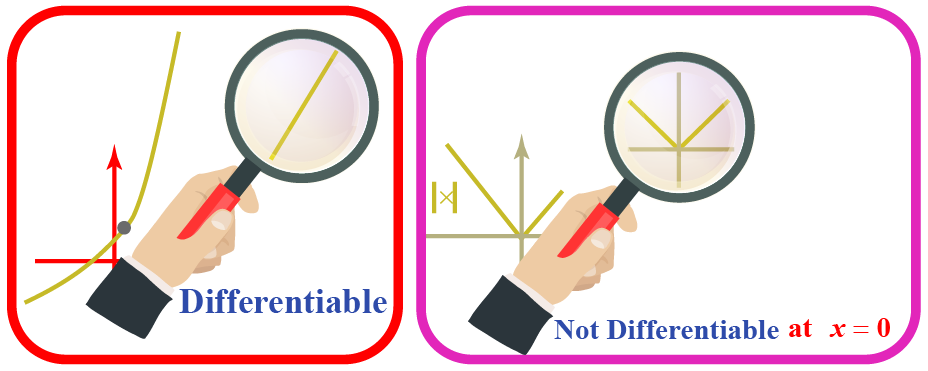What Is the Difference Between Differentiable and Continuous Function?

We say that a function is continuous at a point if its graph is unbroken at that point.

A differentiable function is always a continuous function but a continuous function is not necessarily differentiable.

Example

We already discussed the differentiability of the absolute value function.

By looking at the graph of the function can you check if there is any point of discontinuity?

Clearly, there are no breaks in the graph.

The function is continuous everywhere.

Particularly, the function is continuous at $$x=0$$ but not differentiable at $$x=0$$.

Explore the continuity and differentiability of a function using the simulation given below.

## Solved Examples

Let's look at a few differentiability problems.

 Example 1

Ms. Amy asked her students to find out where the given function is not differentiable using graph and limit definition.Can you determine the required point?

Solution

Let's look at the graph of the function $$f(x)=|x+2|$$.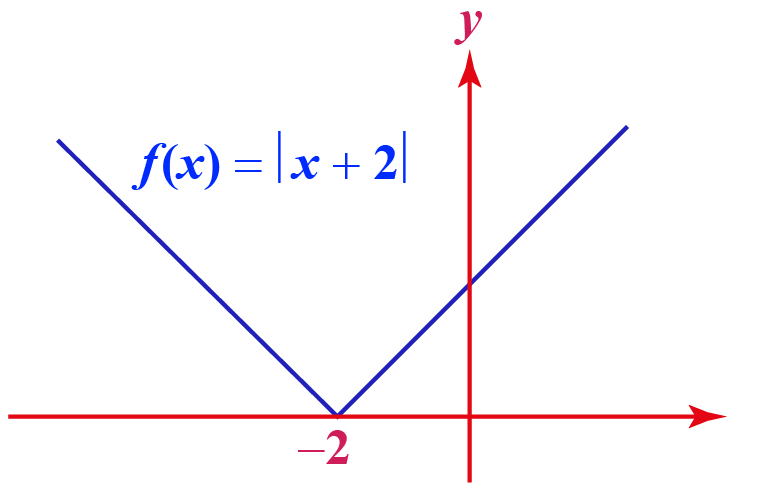Clearly, there is a sharp corner at point $$x=-2$$.

The function is not differentiable at $$x=-2$$.

Now, let's use the limit definition of differentiable functions.

We already observed that the limits are different. \begin{align}\lim_{h\rightarrow 0}\dfrac{f(c+h)-f(c)}{h}&=\lim_{h\rightarrow 0}\dfrac{f(-2+h)-f(-2)}{h}\\&=\lim_{h\rightarrow 0}\dfrac{|h|-0}{h}\\&=\lim_{h\rightarrow 0}\dfrac{|h|}{h}\end{align}

This means that the limit \begin{align}\lim_{h\rightarrow 0}\dfrac{f(c+h)-f(c)}{h}\end{align} does not exists at $$c=-2$$ for $$f(x)=|x+2|$$.

 $$\therefore$$ The point where the function is not differentiable is $$x=-2$$.
 Example 2

Discuss the continuity and differentiability of the function $$g(x)=x^{\frac{1}{3}}$$.

### Solution

Look at the graph of the function $$g(x)=x^{\frac{1}{3}}$$.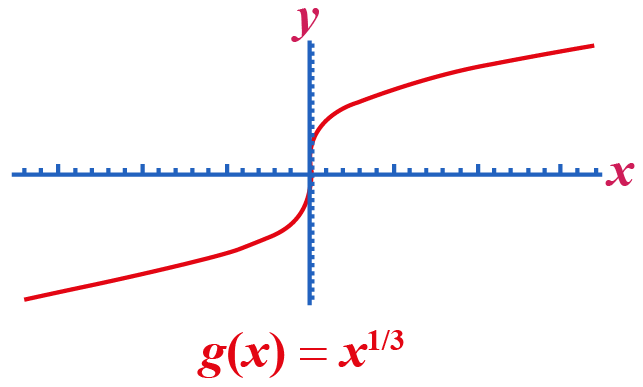The graph is continuous in the coordinate plane.

Observe that the graph has a vertical tangent line at $$x=0$$.

This means that the function is not differentiable at this point.

Let's verify this using the limit definition.

\begin{align}\lim_{h\rightarrow 0}\dfrac{f(c+h)-f(c)}{h}&=\lim_{h\rightarrow 0}\dfrac{f(0+h)-f(0)}{h}\\&=\lim_{h\rightarrow 0}\dfrac{h^{\frac{1}{3}}}{h}\\&=\lim_{h\rightarrow 0}\dfrac{1}{h^{\frac{2}{3}}}\end{align}

This limit does not exist.

 $$\therefore$$ The function $$g(x)=x^{\frac{1}{3}}$$ is continuous but not differentiable at the point $$x=0$$.
 Example 3

Use the derivative rules to find the derivative of $$h(x)=\dfrac{2x+1}{x^3}$$

Solution

We will use the third derivative rule to find the derivative of $$h(x)$$.

\begin{align}\dfrac{dh}{dx}&=\dfrac{d}{dx}\left(\dfrac{2x+1}{x^3}\right)\\&=\dfrac{(2x+1)'x^3-(x^3)'(2x+1)}{(x^3)^2}\\&=\dfrac{2x^3-3x^2(2x+1)}{x^6}\\&=-\dfrac{(4x^3+3x^2)}{x^6}\end{align}

 $$\therefore$$ The derivative of the function $$h(x)=\dfrac{2x+1}{x^3}$$ is $$-\dfrac{(4x^3+3x^2)}{x^6}$$.

Do you want to determine if the function is differentiable by just looking at its graph?

Don't worry! Here are some tricks you can remember while viewing a graph of a function.Tips and Tricks
 1. If a graph has a sharp corner at a point, then the function is not differentiable at that point. 2. If a graph has a break at a point, then the function is not differentiable at that point. 3. If a graph has a vertical tangent line at a point, then the function is not differentiable at that point.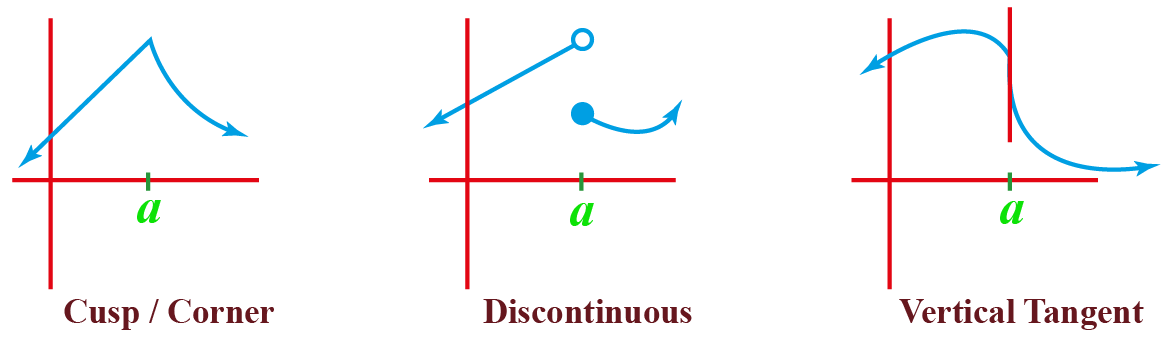## Interactive Questions

Here are a few activities for you to practice.

## Let's Summarize

We hope you enjoyed learning about differentiable with the examples and practice questions. Now, you will be able to easily solve differentiability problems.

At Cuemath, our team of math experts is dedicated to making learning fun for our favorite readers, the students!

Through an interactive and engaging learning-teaching-learning approach, the teachers explore all angles of a topic.

Be it worksheets, online classes, doubt sessions, or any other form of relation, it’s the logical thinking and smart learning approach that we, at Cuemath, believe in.

## 1. Is the cubic function differentiable?

Yes, the cubic function is differentiable.

For example, the function $$f(x)=x^3$$ is differentiable and its derivative is $$f'(x)=3x^2$$.

## 2. What does twice differentiable mean?

If a function is twice differentiable, then it means that the second derivative of the function exists.

## 3. Why is the absolute value function not differentiable at 0?

The absolute value function is not differentiable at 0 because the graph of the function has a sharp corner at this point.

More Important Topics
Numbers
Algebra
Geometry
Measurement
Money
Data
Trigonometry
Calculus